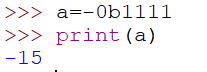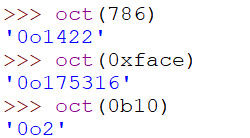top of page• # Data Type - Int

• Representation of 'Int' in different forms - Decimal, Binary, Octal, Hexa Decimal
• # Utility Functions for base conversion

Integer (Int)

it is used to represent integral (whole) values. Numbers without decimal point are known as 'int' like 10, 20, 100 or 1000000 etc. For example:means it is an object of class 'int'.

• In Python-2 'long' is available but in Python-3 long data type is not available.

• We can represent long values also using 'int' variable only.

In 'int' we can represent values in 4 ways:

1.Decimal form

2.Binary form

3.Octal form

4.Hexa decimal form

1. Decimal form: (Base 10)

In decimal form, allowed digits are 0-9.

For example, a = 7878; by default Python is going to consider as decimal form.

2. Binary form: (Base 2)

In binary form, allowed digits are 0 and 1 only.

For example a = 1111Python is going to consider it decimal number only. To let Python consider it as binary, we have to use 0b (zero small b) or 0B (zero capital B).

a = 0b1111 or a = 0B1111To represent negative values

a=-0b1111 or -0B11113. Octal form: (Base 8)

In octal form, allowed digits are 0 to 7.

For example, a = 777

Python is going to consider it decimal number only. To let python consider it as octal we have to use 0o (zero small o) or 0O (zero capital O).4. Hexa decimal form: (Base-16)

In hexa-decimal form, allowed digits are 0 to 9, a to f or A to F. To convey Python consider an input as hexa-decimal, we have to use 0x (zero small x) or 0X (zero capital X).Let us summarize all 4 types with an example.

x=10 (by default Python consider it as decimal)x=0b10 (convey Python consider it as binary)x=0o10 (convey Python consider it as octal)x=0x10 (convey Python consider it as hexa decimal)Utility functions for Base conversion

If you want to change one base to another base.

• bin(): from any base  to binary

• oct(): from any base  to octal

• hext(): from any base to hexadecimal

bin(x): x can be decimal, octal or hexadecimal the corresponding binary value we will get.oct(x): x can be decimal, octal or hexadecimal the corresponding octal value we will get.hex(x): x can be decimal, octal or hexadecimal the corresponding hexa decimal value we will get.

For any Query/Suggestion, do let us know in the comment section.

bottom of page